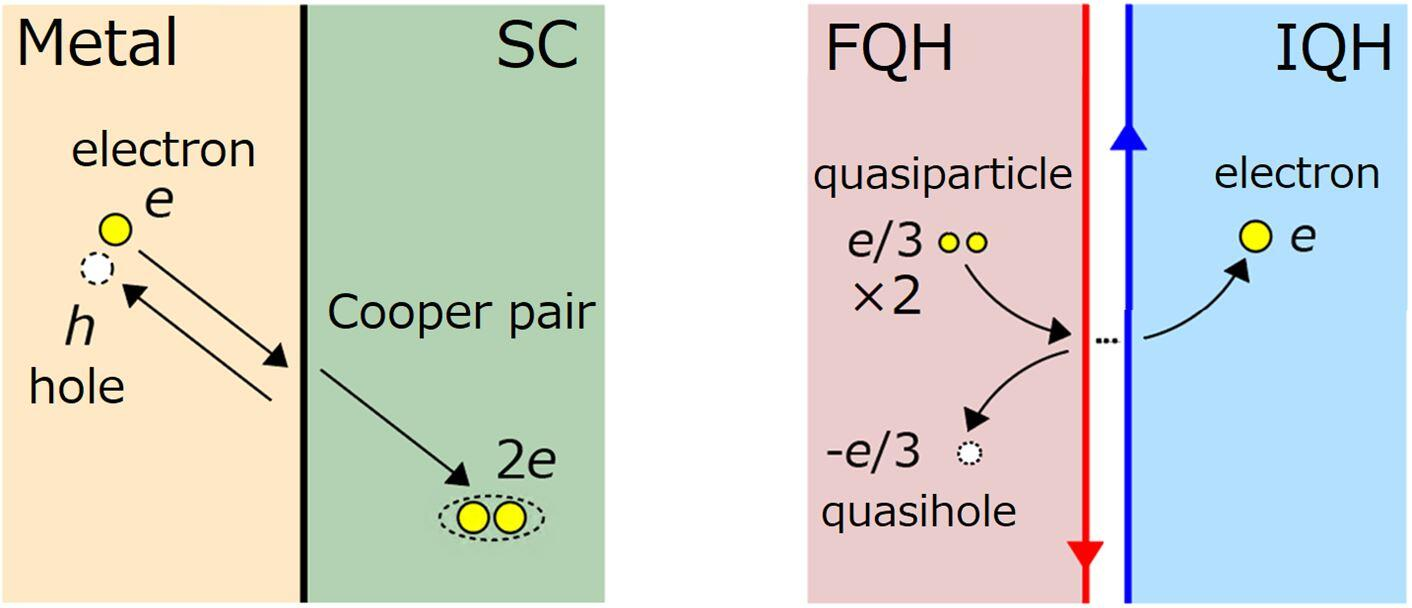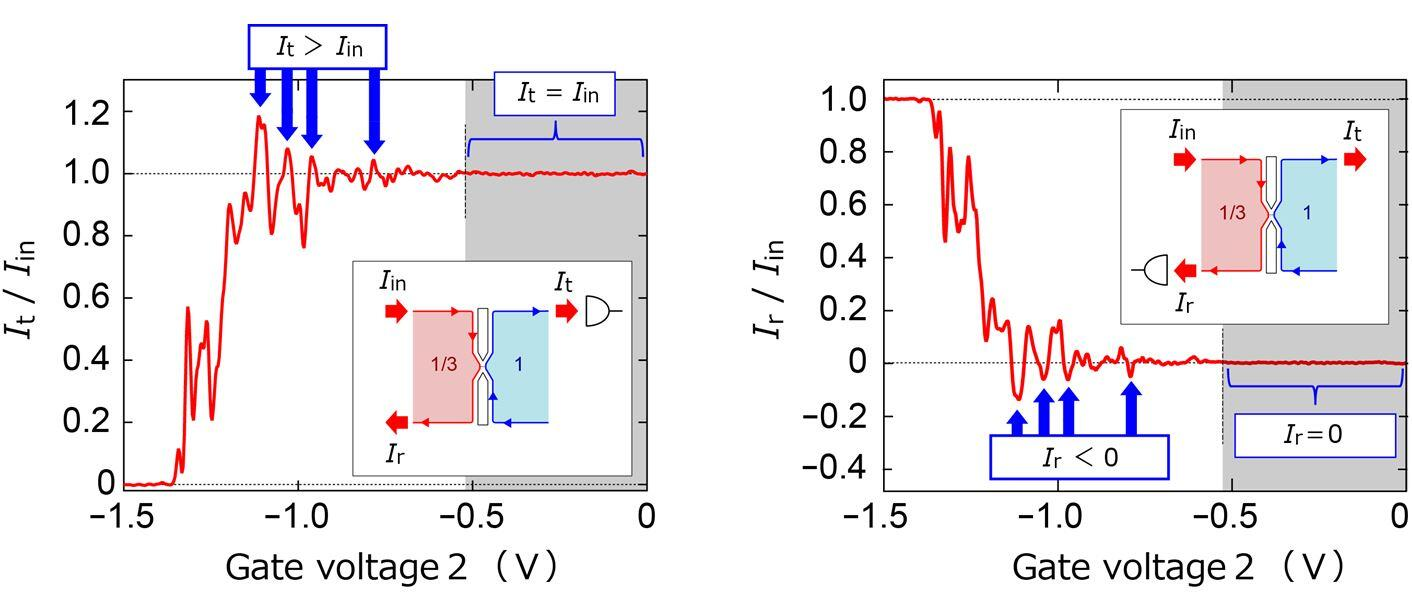Microsoft ends support for Internet Explorer on June 16, 2022.
We recommend using one of the browsers listed below.

May 14, 2021

Nippon Telegraph and Telephone Corporation
Japan Science and Technology Agency

# Andreev reflection of fractionally charged quasiparticles-First observation of Andreev reflection in a material other than superconductors-

### Overview

Nippon Telegraph and Telephone Corporation (NTT) and Japan Science and Technology Agency (JST), in cooperation with the French National Center for Scientific Research [Centre national de la recherche scientifique (CNRS)] and Aix-Marseille University (Aix-Marseille Université (AMU)), have successfully observed Andreev reflection*1 of a fractionally charged quasiparticle*2, a smaller electrical charge than a single electron charge, in a semiconductor. The Andreev reflection occurs at an interface of a fractional quantum Hall state*3 of a two-dimensional electron system*4 in a strong magnetic field at low temperatures. In this phenomenon, the incidence of fractionally charged quasiparticles on an interface transmits an electron and reflects a hole having a fractional charge. Andreev reflection has been considered to be a phenomenon unique to superconductors, where electrons form a pair (Cooper pair) to flow as supercurrent. When an electron is incident on a superconductor, a pair of electrons is transmitted, and a hole is reflected to conserve charge. Theories predicted the Andreev reflection in materials other than superconductors in the 1990s, but no such reflection came to be experimentally observed for more than two decades. Researchers at NTT and JST observed a new type of Andreev reflection that occurs in semiconductors and for fractional charges, which indicates the universality of the Andreev-type reflection mechanism. Certain classes of fractionally charged quasiparticles are expected to find applications in topological quantum computing*5. The experiment clarifies the charge transport mechanism at the interface between a fractional quantum Hall state and normal conductors, providing valuable knowledge for developing a quantum information device to manipulate quasiparticles.

These results were published online in the British scientific journal Nature Communications on May 14, 2021.

### 1. Background

In a superconductor, electrons form a pair (Cooper pair) to flow as supercurrent. When an electron (having negative charge e*6) is incident on a superconductor from the outside, it forms a Cooper pair (charge 2e) to flow into the superconductor, which increases the current. At the same time, as the total amount of charge must be conserved in the overall process (charge conservation law*7), a hole is reflected off the interface to compensate for this increase in transmitted current (Fig. 1, left). This phenomenon is referred to as Andreev reflection, an elementary process*8 of charge transfer at a superconductor interface and a fundamental principle in various devices having a superconductor junction.

Andreev reflection has traditionally been thought of as a phenomenon unique to superconductors. However, underlying this phenomenon is the charge conservation law of broader universality. Therefore, if a charge carrier is not an electron, a similar Andreev-type process may occur for other than superconductors. The fractional quantum Hall state is typical of such states. Applying a strong magnetic field perpendicular to a two-dimensional electron system at low temperatures causes the fractional quantum Hall effect, where Hall conductance quantizes to several rational fractional values. In fractional quantum Hall systems, fractionally charged quasiparticles (referred to below as "quasiparticles"), each having a charge (such as e/3 or e/5) less than that of an electron, carry current. Suppose that quasiparticles are incident on the normal conductor from the fractional quantum Hall system. They flow into the normal conductor as electrons, increasing which increases the transmitted current. At the same time, fractional quasiholes are reflected to satisfy charge conservation, generating a reflected current of opposite sign (Fig. 1, right). This phenomenon manifests that Andreev-type reflection occurs in materials other than superconductors and is the universal charge transport mechanism at the interface between two different types of materials. However, while theories predicted the Andreev process of quasiparticles in the 1990s, it has not been observed for more than two decades because fabricating a clean interface between a fractional quantum Hall state and a normal conductor is difficult. The observation of Andreev reflection that captures the fundamental dynamics of quasiparticles has been a major experimental problem in topological quantum information technology.

The researchers at NTT and JST fabricated a fine fractional-integer quantum Hall junction*9 using nanoscale semiconductor-processing technology and successfully observed the Andreev reflection of quasiparticles.

### 2. Results

The magnetic field applied perpendicular to a two-dimensional electron system in a GaAs semiconductor at low temperatures is adjusted so that the entire electron system would enter an integer quantum Hall state with a Landau-level filling factor*10 of 1. Then, gate voltage 1 is applied to surface gate electrode 1 to decrease the electron density underneath and form a fractional quantum Hall state with a Landau-level filling factor of 1/3, where fractional quasiparticles (e/3) carry current (red region in Fig. 2). At this time, an interface forms inside the sample between the fractional quantum Hall state and integer quantum Hall state (blue region; a state where electrons carry current). In addition, negative gate voltage 2 is applied to fine gate 2 to narrow the interface to less than 1 μm and form a fine fractional-integer quantum Hall junction (Fig. 2). In the experiment, current is driven into this junction from the fractional side and the transmitted current flowing out from the integer side is measured. In doing so, the researchers observed that the transmitted current exceeds the input current at some values of gate voltage 2 (Fig. 3, left). At the same time, they observed the reflected current of the opposite sign to the input current (Fig. 3, right). This increase in transmitted current accompanied by a negative reflected current indicates that Andreev reflection of quasiparticles has occurred. This result shows that Andreev reflection is not limited to superconductors but rather a universal phenomenon.

### 3. Technical points

By using gate electrodes, the researchers realized a fine junction between two distinct quantum Hall states (different Landau-level filling factors, namely, 1/3 and 1) inside the same semiconductor device, rather than at the physical boundary between different materials (Fig. 2). This approach provided a further advantage in that they are also able to precisely control the size of the junction in situ by adjusting the voltages applied to the gates. The fine junction with good quality and high controllability enabled the researchers to observe the Andreev reflection of quasiparticles.

### 4. Future developments

Andreev reflection in superconductors is a fundamental mechanism of various superconductor-based hybrid devices. The Andreev reflection of quasiparticles as reported here shows the potential for fractional quantum Hall effect devices with various new functions.

### 5. Acknowledgments

This work was supported by Grants-in-Aid for Scientific Research (Grant nos. JP16H06009, JP15H05854, JP26247051, and JP19H05603) and JST PRESTO Grant no. JPMJPR1766.

### 6. Publication Details

 Journal: Nature Communications Title: "Andreev reflection of fractional quantum Hall quasiparticles" Authors: Masayuki Hashisaka, Thibaut Jonckheere, Takafumi Akiho, Satoshi Sasaki, Jerome Rech, Thierry Martin, and Koji Muraki DOI: 10.1038/s41467-021-23160-6

### 7. Glossary of terms

*1Andreev reflection
Electric current is the flow of charge carriers. The charge carrier in a normal metal is an electron (each having negative charge e*6) and that in a superconductor is an electron pair (Cooper pair: charge 2e) in a superconductor. When an electron is incident on a superconductor, a Cooper pair forms to increase transmitted current and, at the same time, a hole is reflected to satisfy charge conservation. This phenomenon is referred to as Andreev reflection.

*2Fractionally charged quasiparticle
The minimum unit of excitation carrying electrical charge in a fractional quantum Hall state*3. It has a fractional charge (in this experiment, e/3) smaller than that of an electron. Theories predict that a certain type of quasiparticle in a fractional quantum Hall state will be a building block of a quantum bit in topological quantum computing*5.

*3Quantum Hall state
Applying a strong magnetic field perpendicular to a two-dimensional electron system at low temperatures results in the formation of an electron state characterized by integer values (integer quantum Hall effect) or fractional values (fractional quantum Hall effect) of Hall conductivity in units of e2/h (where h is Planck's constant). In a quantum Hall state, charge carriers flow along a unidirectional one-dimensional channel (edge channel) formed at the edge of the state.

*4Two-dimensional electron system
An electron system confined to a plane, for example, at the interface of a semiconductor heterostructure and in atomic layer material such as graphene.

*5Topological quantum computing
A method of quantum computation using a topological system. It is considered to have a very low error rate, so that is being actively studied worldwide.

*6Negative charge e
The charge of a single electron (e = -1.602×10-19 C). Its absolute value |e| is called the elementary charge, i.e., the smallest unit of charge that can be extracted from a matter.

*7Charge conservation law
A basic law stating that the total amount of charge before and after a physical phenomenon is unchanged.

*8Elementary process
A basic process making up a physical phenomenon. A complex physical phenomenon is expressed as a combination of elementary processes.

*9Fractional-integer quantum Hall junction
A junction connecting a fractional quantum Hall region and an integer quantum Hall region.

*10Landau-level filling factor
The ratio of electron density to a magnetic field (magnetic flux density). An integer quantum Hall effect occurs as this value approaches an integer value and a fractional quantum Hall effect occurs as it approaches a specific fractional value (such as 1/3).

### 8. FiguresFig. 1 Conceptual diagram of Andreev reflection

Left:
Andreev reflection at a conventional metal-superconductor junction
Right:
Andreev reflection of quasiparticles at a fractional-integer quantum Hall (FQH-IQH) junctionFig. 2 Experimental setup

Left:
False-color electron micrograph of the sample. The red region is an FQH region with a Landau-level filling factor of 1/3 formed by applying gate voltage 1 to gate 1, the yellow region is gate 2 for narrowing the two-dimensional electron system, and the blue region is an IQH region with a Landau-level filling factor of 1 (gate 3 is fixed to 0 V). In addition, the dark gray regions are where the semiconductor is etched, while the light gray regions correspond to gate electrodes not used in this experiment. An FQH-IQH junction is formed in the central region enclosed by the green circle. In a quantum Hall region, current flows along unidirectional edge channels (indicated by the arrows in the figure, allowing us to measure both the transmitted and reflected current.
Right:
Conceptual diagram of the FQH-IQH junction (region enclosed by the green circle in the left figure). When applying gate voltage 2 to deplete the underlying two-dimensional electron system, a junction with a width of less than 1 μm is formed at which the fractional and integer regions make contact. The junction width becomes narrower the larger is the negative gate voltage 2.Fig. 3 Transport properties of the FQH-IQH junction

Left:
Transmitted current It measured as a function of gate voltage 2. The vertical axis is normalized by the input current Iin. While It equals Iin when a minute junction is not formed (a gray region in the figure), It oscillates around It/Iin = 1 when a fine junction is formed (gate voltage 2: -0.6 V or less), showing several peaks with It/Iin > 1 (blue arrows).
Right:
Reflected current Ir measured as a function of gate voltage 2. For this plot, we evaluated Ir from the measured voltage. A negative reflected current was observed (blue arrows) simultaneously with an increase in the transmitted current (left part of the figure). The increase in It and negative Ir is evidence of Andreev reflection.

Public Relations Office
NTT Science and Core Technology Laboratory Group
science_coretech-pr-ml@hco.ntt.co.jp
Tel.: 046-240-5157

Public Relations Division
Japan Science and Technology Agency (JST)
jstkoho@jst.go.jp
Tel.: 03-5214-8404

■ Inquiries regarding JST

Green Innovation Group, Department of Innovation Research
Japan Science and Technology Agency (JST)
Yuko Shimabayashi
presto@jst.go.jp
Tel.: 03-3512-3526

Information is current as of the date of issue of the individual press release.